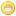simple base conversion formula for tetrationJmsNxnUltimate FellowPosts: 1,214 Threads: 126 Joined: Dec 2010 09/22/2011, 07:41 PM (This post was last modified: 09/22/2011, 07:44 PM by JmsNxn.) Well I finally decided to look a little deeper at my suggested base conversion, and to my surprise, the formula just popped out at me. given the definition $k \ge 0$: $a\,\,\bigtriangleup_{-k}^e\,\, b = \ln^{\circ k}(\exp^{\circ k}(a) + \exp^{\circ k}(b))$ we see instantly: $e^x\,\,\bigtriangleup_{-k}^e\,\,e^y = e^{x\,\,\bigtriangleup_{-k-1}^e\,\,y}$ which works for k = 0 as well since $\bigtriangleup_{1}^e$ is multiplication. It's easy now to generate a formula which works for base conversion using these operators. The notation for such is very cumbersome however, therefore I'll write it out step by step for 2, 3, 4. $b^b = e^{\ln(b) \cdot e^{\ln(b)}} = e^{e^{\ln(b) + \ln(\ln(b))}} = \,\,^{2+\text{slog}_e(\ln(b) + \ln(\ln(b)))}e$ $b^{b^b} = e^{\ln(b) \cdot e^{\ln(b) \cdot e^{\ln(b)}}} = e^{e^{e^{\ln(b) + \ln(\ln(b))\, \bigtriangleup_{-1}^e \,\ln(\ln(\ln(b)))}}}= \,\,^{3+\text{slog}_e(\ln(b) + \ln(\ln(b)) \bigtriangleup_{-1}^e \ln(\ln(\ln(b))))}e$ and which I think the process becomes obvious by this point $b^{b^{b^{b}}} = \,\,^{4 + \text{slog}_e(\ln(b) + \ln(\ln(b)) \,\bigtriangleup_{-1}^e\, \ln(\ln(\ln(b)))\,\bigtriangleup_{-2}^e\,\ln(\ln(\ln(\ln(b)))))} e$ and which by generality becomes: $^k b = \,\,^{k + \text{slog}_e(\ln(b) + \ln^{\circ 2}(b) \,\bigtriangleup_{-1}^e\,\ln^{\circ 3}(b)\,\bigtriangleup_{-2}^e\,\ln^{\circ 4}(b)\,...\,\bigtriangleup_{-k+1}\,\ln^{\circ k-1}(b)\,\bigtriangleup_{-k+2}^e\,\ln^{\circ k}(b))}e$ where we are sure to evaluate the highest operator first. this formula is very easily generalized to include conversion from base $b > \eta$ to any base $c > \eta$ « Next Oldest | Next Newest »

 Possibly Related Threads… Thread Author Replies Views Last Post Simple limit approximation to exp(x) tommy1729 0 260 05/16/2023, 11:13 PM Last Post: tommy1729 [2sinh] exp(x) - exp( - (e-1) x), Low Base Constant (LBC) 1.5056377.. tommy1729 3 662 04/30/2023, 01:22 AM Last Post: tommy1729 f(x+y) g(f(x)f(y)) = f(x) + f(y) addition formula ? tommy1729 1 503 01/13/2023, 08:45 PM Last Post: tommy1729 Base -1 marraco 15 22,357 07/06/2022, 09:37 AM Last Post: Catullus I thought I'd take a crack at base = 1/2 JmsNxn 9 4,118 06/20/2022, 08:28 AM Last Post: CatullusFormula for the Taylor Series for Tetration Catullus 8 3,723 06/12/2022, 07:32 AM Last Post: JmsNxnRepetition of the last digits of a tetration of generic base Luknik 12 6,595 12/16/2021, 12:26 AM Last Post: marcokrt On the $$2 \pi i$$-periodic solution to tetration, base e JmsNxn 0 1,200 09/28/2021, 05:44 AM Last Post: JmsNxn A different approach to the base-change method JmsNxn 0 1,660 03/17/2021, 11:15 PM Last Post: JmsNxn Nixon-Banach-Lambert-Raes tetration is analytic , simple and “ closed form “ !! tommy1729 11 8,261 02/04/2021, 03:47 AM Last Post: JmsNxn

Users browsing this thread: 1 Guest(s)